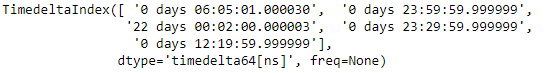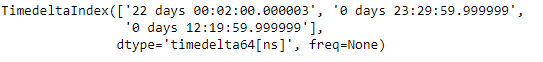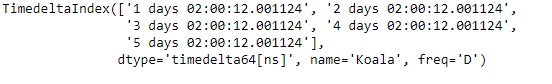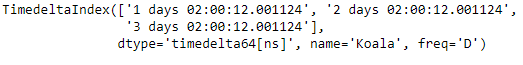# Python | Pandas TimedeltaIndex.take()

• Last Updated : 06 Jan, 2019

Python is a great language for doing data analysis, primarily because of the fantastic ecosystem of data-centric python packages. Pandas is one of those packages and makes importing and analyzing data much easier.

Pandas` TimedeltaIndex.take()` function return a new Index of the values selected by the indices. We generally pass a list of indices to be taken. It is used for internal compatibility with numpy arrays.

Syntax : TimedeltaIndex.take(indices, axis=0, allow_fill=True, fill_value=None, **kwargs)

Parameters :
indices : list Indices to be taken
axis : The axis over which to select values, always 0.
allow_fill : bool, default True
fill_value : bool, default None ,If allow_fill=True and fill_value is not None, indices specified by -1 is regarded as NA. If Index doesn’t hold NA, raise ValueError

Return : Object of same type

Example #1: Use `TimedeltaIndex.take()` function to return a new TimedeltaIndex object containing only selected values in it.

 `# importing pandas as pd``import` `pandas as pd`` ` `# Create the TimedeltaIndex object``tidx ``=` `pd.TimedeltaIndex(data ``=``[``'06:05:01.000030'``, ``'+23:59:59.999999'``, ``                        ``'22 day 2 min 3us 10ns'``, ``'+23:29:59.999999'``, ``                        ``'+12:19:59.999999'``])`` ` `# Print the TimedeltaIndex object``print``(tidx)`

Output :Now we will use the `TimedeltaIndex.take()` function to select some specific values from tidx.

 `# select specific values.``tidx.take([``2``, ``3``, ``4``])`

Output :As we can see in the output, the `TimedeltaIndex.take()` function has returned a new TimedeltaIndex object which contains only those elements whose locations has been passed to the function.

Example #2: Use `TimedeltaIndex.take()` function to return a new TimedeltaIndex object containing only selected values in it.

 `# importing pandas as pd``import` `pandas as pd`` ` `# Create the TimedeltaIndex object``tidx ``=` `pd.TimedeltaIndex(start ``=``'1 days 02:00:12.001124'``, ``                         ``periods ``=` `5``, freq ``=``'D'``, name ``=``'Koala'``)`` ` `# Print the TimedeltaIndex object``tidx`

Output :Now we will use the `TimedeltaIndex.take()` function to select some specific values from tidx.

 `# select specific values.``tidx.take([``0``, ``1``, ``2``])`

Output :As we can see in the output, the `TimedeltaIndex.take()` function has returned a new TimedeltaIndex object which contains only those elements whose locations has been passed to the function.

My Personal Notes arrow_drop_up